Clique Problem

# Clique Problem - Theory of Computation - Computer Science Engineering (CSE)

Part V. Clique Problem

9 Clique Problem

Clique
Let we are given a graph with a number of vertices. A clique is a subset of vertices, such that an edge is present between every pair of vertices.
Consider the following graph: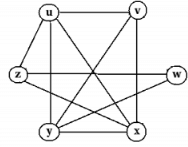In the above graph, a clique is {u,v,x,y}. The size of this clique is 4.
A clique is a subgraph of the above graph which is shown below: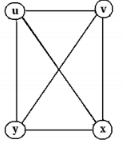The above set is a clique, because uv, ux, uy, vx, vy, xy are edges in the given graph.

Clique Problem
Clique problem is to check whether a clique of size k is present in the graph.

Clique Problem is NP-Complete
We know that a problem is in class NP-Complete if: it is in NP and it is NP Hard.

Theorem:
Clique problem is NP-complete.

Proof:
Step 1: Prove that clique problem is in class NP.
A set with n elements has 2possible subsets. Then a graph with n vertices has 2n possible subgraphs. So an algorithm needs to check whether any one of these subgraphs form a clique. It has worst case time complexity Θ(2n). (not polynomial time, but exponential time). Let we are given a graph and a ’certificate’ telling that a subset of vertices form a clique. An algorithm can verify this in polynomila time. So clique problem is in class NP.

Step 2: Prove that clique problem is NP-hard.
We know that a problem is NP-Hard, if every problem in NP can be reduced to this problem in polynomial time. The approach we used is as follows:
In the previous section, we already proved that all NP problems can be reduced to SAT. Then, again we found that SAT problem can be reduced to 3-CNF-SAT problem. Then, if we can reduce 3-CNF-SAT problem to clique problem in polynomial time, we can say that clique is NP-hard.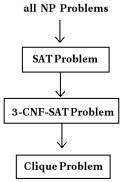We need to reduce 3-CNF-SAT problem to clique problem.
Consider a 3-CNF boolean formula given below:
(a ∪ ¬b ∪ ¬c) ∩ (¬a ∪ b ∪ c) ∩ (a ∪ b ∪ c)
A graph is produced from the above formula as: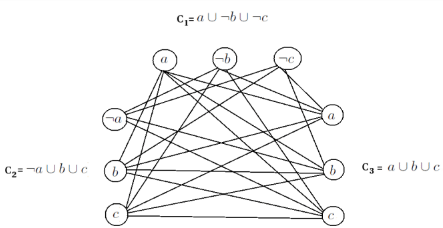The graph is produced as follows:
Every vertex in a clause is connected to every other vertex in another clause. But a vertex x should not be connected to vertex x.
This graph corresponding to 3-CNF-SAT has a solution if the corresponding vertices form a clique.
In the above graph, vertices ¬b of C1, ¬a of C2, c of C3 form a clique.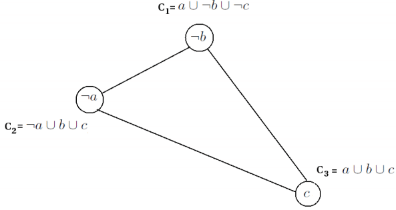In the above clique, Chas the vertex ¬b. So put b=0.
C2 has the vertex ¬a. So put a=0.
C3 has the vertex c. So put c=1.

Thus a satisfying assignment to the given boolean formula is a=0, b=0, c=1.
Consider the given boolean formula,
(a ∪ ¬b ∪ ¬c) ∩ (¬a ∪ b ∪ c) ∩ (a ∪ b ∪ c)
Put a=0, b=0, c=1, we get,
(0 ∪ ¬0 ∪ ¬1) ∩ (¬0 ∪ 0 ∪ 1) ∩ (0 ∪ 0 ∪ 1)
=(0 ∪ 1 ∪ 0) ∩ (1 ∪ 0 ∪ 1) ∩ (0 ∪ 0 ∪ 1)=1 ∩ 1 ∩ 1=1
Thus the given formula in 3-CNF gives true for a=0, b=0, c=1.

Thus the given 3-CNF-SAT problem is reduced to clique problem. This means clique problem is NP-hard.

In step 1, we proved that clique is in NP.
In step 2, we proved that clique is NP-hard.
So, clique is an NP-complete problem.

The document Clique Problem | Theory of Computation - Computer Science Engineering (CSE) is a part of the Computer Science Engineering (CSE) Course Theory of Computation.
All you need of Computer Science Engineering (CSE) at this link: Computer Science Engineering (CSE)

## Theory of Computation

18 videos|56 docs|44 tests

## FAQs on Clique Problem - Theory of Computation - Computer Science Engineering (CSE)

 1. What is the clique problem in computer science engineering?Ans. The clique problem in computer science engineering refers to a computational problem where the task is to find a subset of vertices in an undirected graph such that every pair of vertices in the subset is connected by an edge.
 2. How is the complexity of the clique problem determined?Ans. The complexity of the clique problem is determined by the size of the largest clique in the given graph. Finding the largest clique in a graph is known to be an NP-complete problem, which means it is computationally difficult to solve efficiently for large graphs.
 3. Can the clique problem be solved using brute force?Ans. The clique problem can be solved using a brute force approach, where all possible subsets of vertices are checked for being cliques. However, this approach has an exponential time complexity, making it impractical for large graphs.
 4. Are there any approximation algorithms for the clique problem?Ans. Yes, there are approximation algorithms for the clique problem that provide a solution that is guaranteed to be close to the optimal solution. One such algorithm is the vertex cover algorithm, which finds a subset of vertices that covers all the edges in the graph.
 5. What are some applications of the clique problem in computer science engineering?Ans. The clique problem has various applications in computer science engineering, including social network analysis, computer vision, data mining, and bioinformatics. For example, in social network analysis, cliques can represent groups of individuals with strong connections, while in bioinformatics, cliques can represent genes or proteins that interact with each other.

## Theory of Computation

18 videos|56 docs|44 testsExplore Courses for Computer Science Engineering (CSE) examSignup to see your scores go up within 7 days! Learn & Practice with 1000+ FREE Notes, Videos & Tests.
10M+ students study on EduRev
Track your progress, build streaks, highlight & save important lessons and more!
Related Searches

,

,

,

,

,

,

,

,

,

,

,

,

,

,

,

,

,

,

,

,

,

;